# MHD Equations¶

## Introduction¶

The magnetohydrodynamics (MHD) equations are:

(1)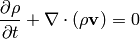(2)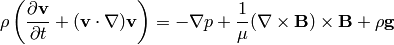(3)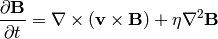(4)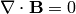assuming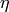is constant. See the next section for a derivation. We can now apply the following identities (we use the fact that):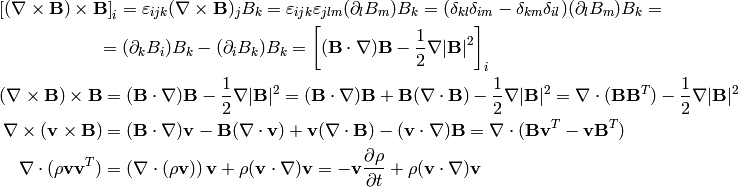So the MHD equations can alternatively be written as:

(5)(6)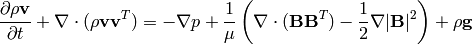(7)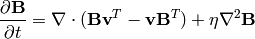(8)One can also introduce a new variable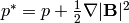, that simplifies (6) a bit.

## Derivation¶

The above equations can easily be derived. We have the continuity equation:Navier-Stokes equations (momentum equation) with the Lorentz force on the right-hand side: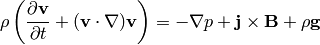where the current densityis given by the Maxwell equation (we neglect the displacement current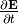):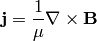and the Lorentz force: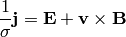from which we eliminate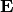: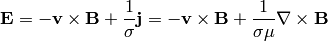and put it into the Maxwell equation: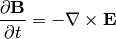so we get: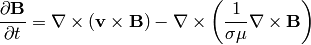assuming the magnetic diffusivity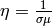is constant, we get: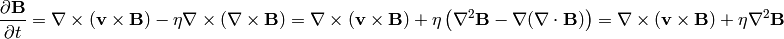where we used the Maxwell equation:## Finite Element Formulation¶

We solve the following ideal MHD equations (we use, but we drop the star):

(9)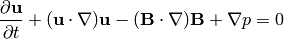(10)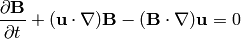(11)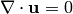(12)If the equation (12) is satisfied initially, then it is satisfied all the time, as can be easily proved by applying a divergence to the Maxwell equation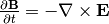(or the equation (10), resp. (3)) and we get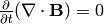, so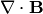is constant, independent of time. As a consequence, we are essentially only solving equations (9), (10) and (11), which consist of 5 equations for 5 unknowns (components of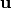,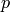and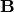).

We discretize in time by introducing a small time step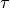and we also linearize the convective terms:

(13)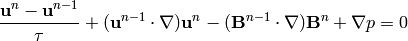(14)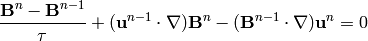(15)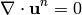Testing (13) by the test functions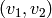, (14) by the functions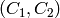and (15) by the test function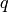, we obtain the following weak formulation:

(16)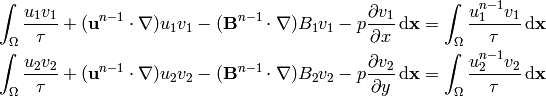(17)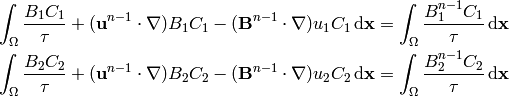(18)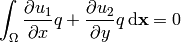To better understand the structure of these equations, we write it using bilinear and linear forms, as well as take into account the symmetries of the forms. Then we get a particularly simple structure: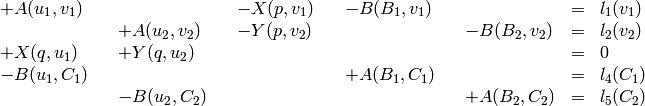where: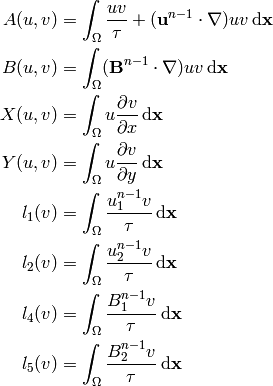E.g. there are only 4 distinct bilinear forms. Schematically we can visualize the structure by:

 A -X -B A -Y -B X Y -B A -B A

In order to solve it with Hermes, we first need to write it in the block form: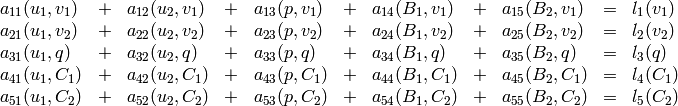comparing to the above, we get the following nonzero forms: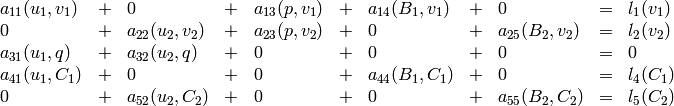where: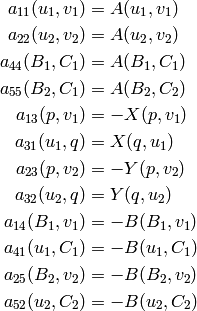and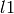, ...,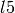are the same as above.